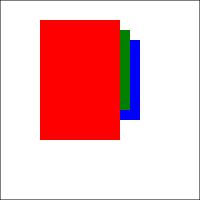# Layout#

Elsie contains a layout system which allows you to quickly build scenarios that are common in presentations, while still providing the option of finely tuned customization for situations where every pixel placement matters.

The central element of the layout system is the Box.

Each Elsie slide contains a layout hierarchy tree. The internal nodes of the tree are boxes and the leaves are box items. Boxes are layout containers, which do not produce any visual content, but they dictate how are their children laid out on a slide. Box items are individual paintable items, such as text, images, shapes, etc. Anything that can be rendered by Elsie thus has an accompanied parent box which decides its size and position on a slide.

## Creating boxes#

To create a new box, you can call the `box` method on an existing `Box`. This will return a new box which will be a child of the box object on which you call the `box` method. The root box of the slide layout hierarchy is available to you as the return value of the `new_slide` method or the `slide` decorator.

Here we create three boxes as children of the top-level slide box and create a child text item in each box.

``````slide.box().text("Box 1")
slide.box().text("Box 2")
slide.box().text("Box 3")
``````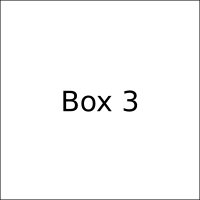Note: for brevity, most code snippets in this user guide assume that there is a `slides` variable containing a `SlideDeck` object and a `slide` variable containing a `Slide` object. The `render` method call is also omitted from most of the examples that display rendered slide output. The output from the code snippets is rendered into PNG images instead of SVG images to ensure that they will be displayed consistently on each device.

### Debug draw mode#

The boxes themselves are invisible, but they have caused the three text items to be rendered below one another. If you are fine-tuning or debugging the layout of your slide, and you want to see the extents and bounds of your boxes, you can use the `debug_boxes` parameter when creating a slide:

``````@slides.slide(debug_boxes=True)
def three_boxes_debug(slide):
slide.box().text("Box 1")
slide.box().text("Box 2")
slide.box().text("Box 3")
``````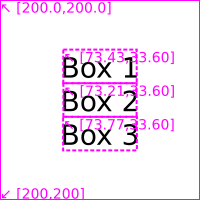You can see that there is a single root box that wraps the whole slide and then there are three individual boxes in the middle. The box debug draw mode will be used in some examples on this page to demonstrate the extents of individual boxes.

### Default box layout properties#

Newly created boxes have the following behavior by default:

• They occupy as few space as possible. You can change this by modifying their size.
• Children are placed vertically in a column, in the order in which they were created. You can change this by modifying their axis.
• Children are centered vertically and horizontally. You can change this by modifying their position.

In the following sections below we will see how these default positioning and sizing rules can be changed.

### Box naming#

Boxes can be named with the `name` parameter (`.box(name="My box")`). It has no impact on normal rendering of the slide, but the name will be shown if the box debug draw mode is enabled.

If you use the `@slides.slide()` decorator, the name of the top-level slide will be set to the name of the function on which the decorator was used.

## Box axis#

Boxes can either be vertical or horizontal:

• Vertical boxes place its child items vertically in a column. Their main axis is vertical and their cross axis is horizontal.
• Horizontal boxes place its child items horizontally in a row. Their main axis is horizontal and their cross axis is vertical.

Boxes are vertical by default, if you want to create a horizontal box, use the `horizontal=True` parameter when creating a box:

``````box = slide.box(horizontal=True)
box.box().text("Box 1")
box.box().text("Box 2")
``````## Composing boxes#

By composing boxes, you can create complex hierarchical row and column layouts.

``````row = slide.box(horizontal=True)
col_a = row.box()
col_a.box().text("Col. A/1")
col_a.box().text("Col. A/2")
col_b = row.box()
col_b.box().text("Col. B/1")
``````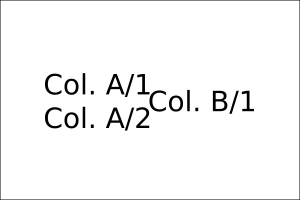Note that almost all methods on a box will create a new box (`box`) or an item (e.g. `text` or `rect`). Leaf items cannot contain children, but for convenience they also offer most of the methods available on boxes, which they delegate to their parent. Therefore, the following two snippets will create the same slide content:

``````# A: create text on its parent box
box = slide.box()
box.rect(bg_color="#aaf")
box.text("Hello!")

# B: create text on its sibling rectangle
slide.box().rect(bg_color="#aaf").text("Hello!")
``````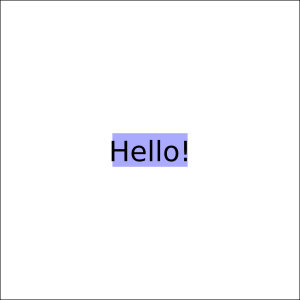## Sizing boxes#

You can change the width and height of a box by using the `width` and `height` parameters of the `box` method.

Here we create three boxes (`A`, `B` and `C`). Box `A` has a default size, which is set according to the required size of its text child. Box `B` has a width of `300` pixels and height of `100` pixels. Box `C` has width equal to the full width of its parent and height is again set to the height of its child text item.

``````slide.box(name="A").text("Box 1")
slide.box(name="B", width=300, height=100).text("Box 2")
slide.box(name="C", width="100%").text("Box 3")
``````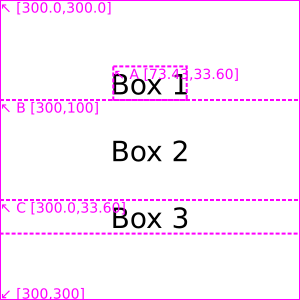The `width` and `height` parameters define the minimal size of the box. Therefore, if its children (child boxes, text items, etc.) request a larger size, the box will use the requested size of its content.

### Size value formats#

You can enter `width` and `height` values in several formats:

• `None` (the default): No minimal size request.
• `int`, `float` or a string containg only digits: Exact minimal size defined in pixels.
• `"<number>%"` (e.g. `"50%"`): Minimal size in percentage of the parent box size.
• `"fill"` or `"fill(<number>)"`: The box will fill all available space of the parent box. If there are more boxes on the same level which use fill, then the size will be distributed amongst them, while respecting the ratio of the `fill` parameter. For example, when one box has argument `fill(2)` and the second one `fill(3)`, the remaining size will be divided using the ratio `2:3`. Using just `"fill"` is a shortcut for `"fill(1)"`.

The following code shows an example of `"fill"` usage:

``````slide.box(width="fill").text("Box 1")
box = slide.box(width=300, height=180)
box.box(height="fill(1)").text("Box 2")
box.box(height="fill(2)").text("Box 3")
slide.box(height="fill").text("Box 4")
``````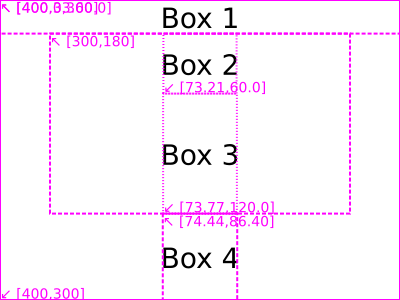### Aliases for commonly sized boxes#

Elsie contains three shortcuts for creating boxes with common minimal size requirements:

• `fbox` (fill-box): shortcut for `box(width="fill", height="fill")`.
• `sbox` (stretch-box): shortcut for `box(width="fill")` if the parent box has a vertical layout or `box(height="fill")` if the parent box has a horizontal layout. In other words, it fills the box in the cross axis.
• `overlay`: shortcut for `box(x=0, y=0, width="100%", height="100%)`. This can be used if you want to overlay several boxes on top of each other, which is useful especially in combination with revealing.

### Padding#

By default, each box gives all of its space to its children. This can be modified by padding. There are four padding values: `left`, `right`, `top`, and `bottom`. They can be modified with the `p_left`, `p_right`, `p_top`, and `p_bottom` parameters of the `box` method.

After the layout of the parent box is computed and the final size and position of a box is known, the padding will shrink its size in the specified directions.

``````slide.box(width=160, height=100, p_left=40, name="Top box")
slide.box(width=160, height=150, p_y=50, name="Bottom box")
``````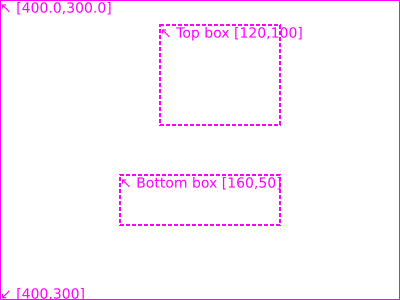In the above example, the top box is shrunk by `40` pixels from the left. Note that the padding is applied after the layout was calculated, therefore the box was first centered horizontally, and then the padding reduced its size. The bottom box is shrunk by `50` pixels from the top and from the bottom.

You can also use the following padding shortcut parameters of the `box` method:

• `p_x` sets both `p_left` and `p_right`.
• `p_y` sets both `p_top` and `p_bottom`.
• `padding` sets all four paddings at once.

## Positioning boxes#

You can set the position of the top-left corner of a box via the `x` and `y` parameters of the `box` method. You can enter the `x` and `y` positions in several formats:

• `None` (default): Set the default position (see below).
• `int`, `float` or a string containing only digits: Set absolute position in pixels. Coordinates are relative to the top-left corner of the parent box.
• `"<number>%`: Set position relative to the parent box. `"0%"` represents the left (`x`) or top (`y`) edge of the parent and `"100%"` the right (`x`) or bottom (`y`) edge of the parent.
• `"[<number>%]`: Align the box in the parent box. `"[0%]"` is left (`x`) or top (`y`), `"[50%]"` is middle and `"[100%]"` is right (`x`) or bottom (`y`) alignment.
• Dynamically defined position: See below.

Here is an example of using absolute and relative position coordinates:

``````slide.box(x=0, y=10).text("Box 1")
row = slide.box(width="fill")
row.box(x="20%").text("Box 2")
row.box(x="60%").text("Box 3")
``````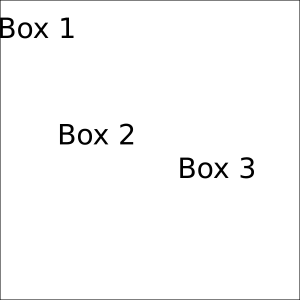### Default position#

The default positioning of a box depends on the axis of its parent. The following explanation assumes a vertical box. For a horizontal box, the main and cross axes would be swapped.

Children of a box will be by default centered along the cross axis. In the case of a vertical parent box, the `x` attribute would be set to `"[50%]"`, i.e. child boxes will be horizontally centered.

For the main axis, the behaviour is more complex. When a box has its main axis position set to `None`, it is a managed box. A parent box stacks all of its managed children boxes along its main axis one-by-one. In addition, it also centers all of its children together along the main axis.

``````slide.box().text("Box 1")
slide.box().text("Box 2")
slide.box().text("Box 3")
``````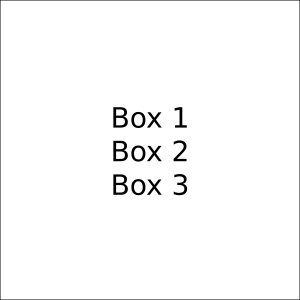In the above example, the `slide` is a parent vertical box. Its three children will thus be laid below one another, they will be centered horizontally, and all of them together will also be centered vertically.

Here is the same situation with a horizontal parent box:

``````row = slide.box(horizontal=True)
row.box().text("Box 1")
row.box().text("Box 2")
row.box().text("Box 3")
``````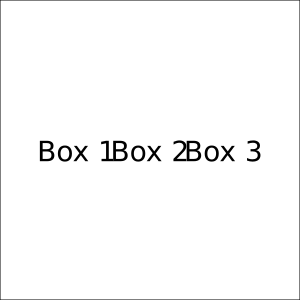### Dynamic positions#

Box position can also be defined dynamically with respect to other boxes. You can get a position that is relative to the final position of a box using the `x` or `y` methods. The returned value of these methods will be a proxy object that will resolve the actual final position after layout is computed.

The reference point of these two methods is the top-left corner of the target box. The parameter can be either:

• `int` or `float`: Resolves to the given number of pixels from the reference point. The value can be negative.
• `"<number>%"`: Resolves to the ratio of the size of the box added to the reference point.

For example:

• `.x("0%")` returns the left-most `x` coordinate of the box.
• `.x("50%")` returns the `x` coordinate of the middle of the box.
• `.x("100%")` return the right-most `x` coordinate of the box.
• `.x(10)` returns the left-most `x` coordinate of the box, moved by `10` pixels to the right.

Here you can observe dynamic positions in action:

``````b = slide.box(width=100, height=100, name="First")
slide.box(x=b.x("50%"), y=b.y("50%"), width=100, height=100, name="Second")
slide.box(x=0, y=b.y("100%"), width="100%", height=200, name="Third")
``````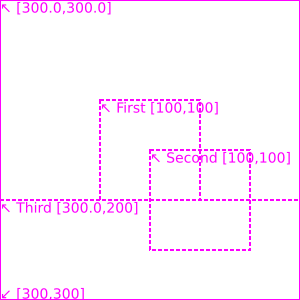In addition to using the individual `x` and `y` methods, you can also use the `p` method to create a dynamic point, which will again be resolved after the layout is fully computed. You can also further move this point via the `add` method. This is mostly useful for defining points of lines and polygons.

## Modifying render order#

By default, boxes are rendered by performing a depth-first walk through the layout tree. Each child is visited in the order in which it was defined.

In the following example, the blue box is rendered over all the previous boxes as it was defined the last.

``````slide.box(x=40, y=20, width=80, height=120).rect(bg_color="red")
slide.box(x=50, y=30, width=80, height=80).rect(bg_color="green")
slide.box(x=60, y=40, width=80, height=80).rect(bg_color="blue")
``````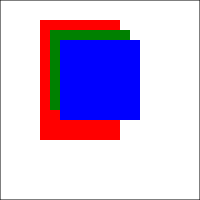You have several options how to change the rendering order:

• Use the `prepend` parameter when creating a box. This will insert it as the first child of the parent box, instead of the last.
``````slide.box(x=40, y=20, width=80, height=120).rect(bg_color="red")
slide.box(x=50, y=30, width=80, height=80).rect(bg_color="green")
slide.box(x=60, y=40, width=80, height=80, prepend=True).rect(bg_color="blue")
``````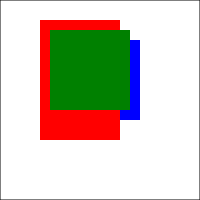• Use the `below` or `above` parameters when creating a box to place the newly created box above/below the box passed in the parameter.
``````a = slide.box(x=40, y=20, width=80, height=120)
a.rect(bg_color="red")
slide.box(x=50, y=30, width=80, height=80, below=a).rect(bg_color="green")
slide.box(x=60, y=40, width=80, height=80).rect(bg_color="blue")
``````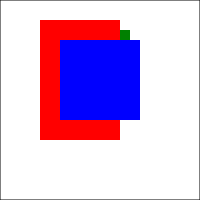• You can also move boxes in the `z` axis using the `z_level` parameter. Before the final paint, all drawing elements will be sorted using a stable sort by their `z_level`. An element with a larger `z_level` will be drawn after an element with a small `z_level`. If the `z_level` is not specified, it is inherited from the parent box. The root box has `z_level` set to `0`.
``````slide.box(x=40, y=20, width=80, height=120, z_level=3).rect(bg_color="red")
slide.box(x=50, y=30, width=80, height=80, z_level=2).rect(bg_color="green")
slide.box(x=60, y=40, width=80, height=80, z_level=1).rect(bg_color="blue")
``````